# Math in Focus Grade 1 Chapter 1 Practice 3 Answer Key Making Number Patterns

This handy Math in Focus Grade 1 Workbook Answer Key Chapter 1 Practice 3 Making Number Patterns detailed solutions for the textbook questions.

## Math in Focus Grade 1 Chapter 1 Practice 3 Answer Key Making Number Patterns

What comes next in each pattern? Write the number.

Question 1.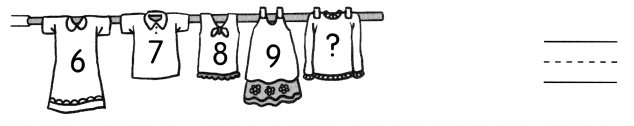Explanation:
The number after 9 is 10
9 + 1 = 10

Question 2.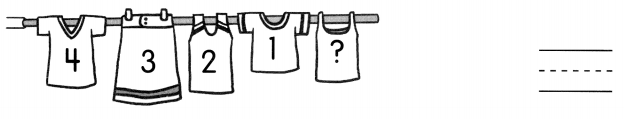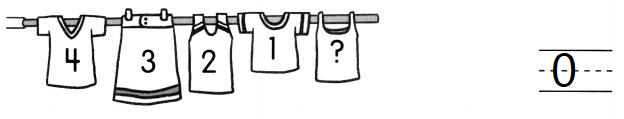Explanation:
The number before 1 is 0.

Question 3.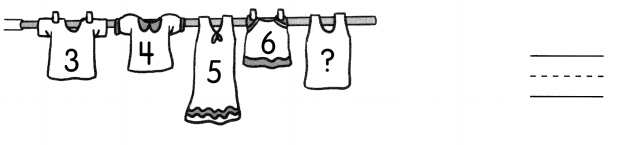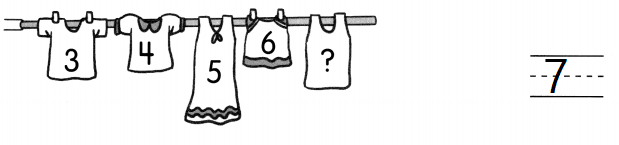Explanation:
The number after 6 is 7
6 + 1 = 7

What is 1 more? Write the number.

Question 4.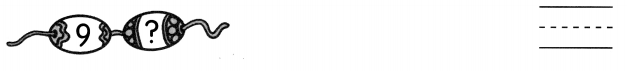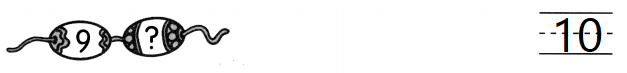Explanation:
The number after 9 is 10
9 + 1 = 10

Question 5.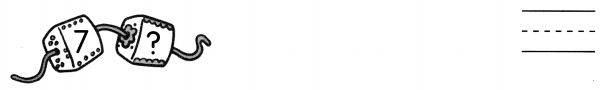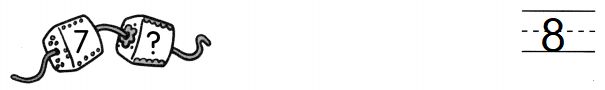Explanation:
The number after 7 is 8
7 + 1 = 8

Question 6.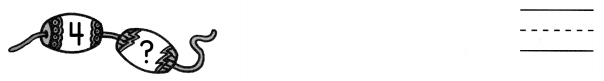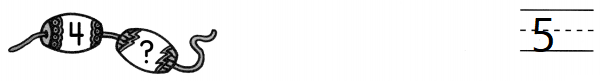Explanation:
The number after 4 is 5
4 + 1 = 5

What is 1 less? Write the number.

Question 7.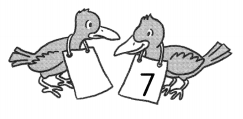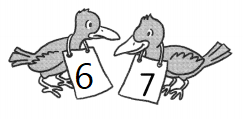Explanation:
The number after 6 is 7
6 + 1 = 7

Question 8.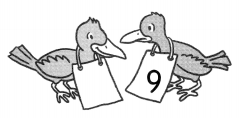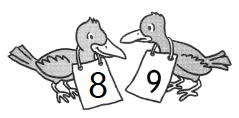Explanation:
The number before 9 is 8
9 – 1 = 8

Question 9.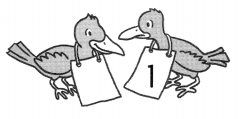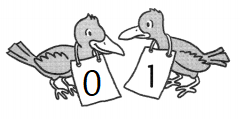Explanation:
The number before 1 is 0.

Write the missing numbers in the number patterns.

Question 10.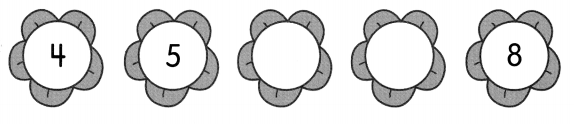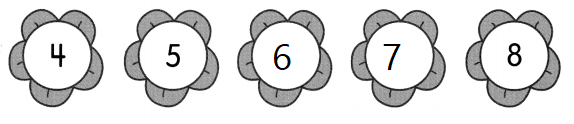Explanation:
The missing numbers are 6 and 7
4, 5 , 6 , 7 , 8.

Question 11.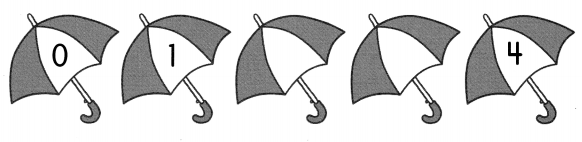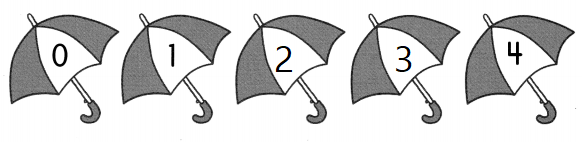Explanation:
The missing numbers are 2 and 3
0, 1 , 2, 3, 4

Write the missing numbers in the number patterns.

Question 12.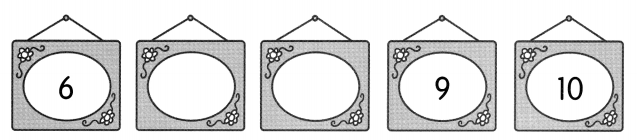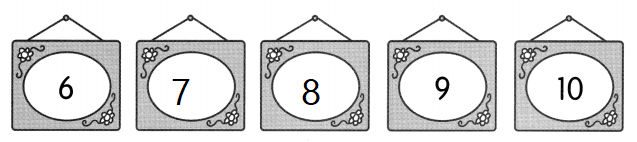Explanation:
In the above pattern 7 and 8 are missing
6, 7, 8 , 9, 10

Question 13.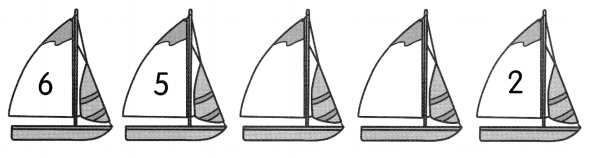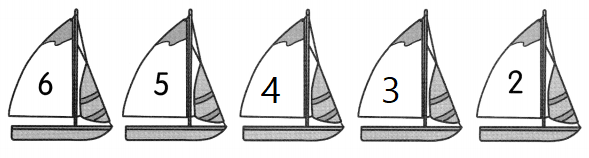Explanation:
In the above pattern 4 and 3 are missing
6, 5, 4, 3, 2, 1.

Question 14.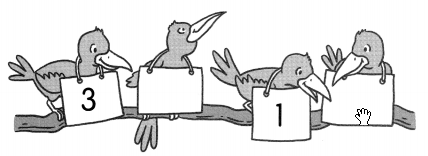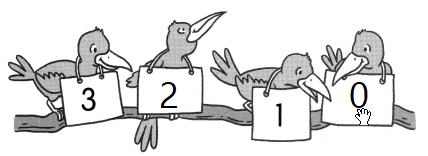Explanation:
In the above pattern 2 and 0 are missing
3, 2, 1, 0

Question 15.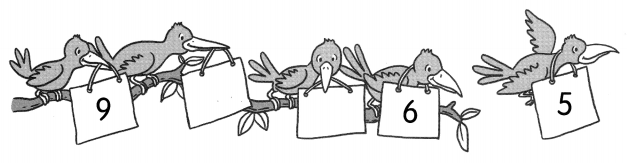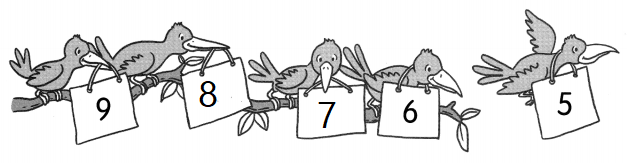Explanation:
In the above pattern 8 and 7 are missing
9, 8 , 7 , 6 , 5.

Fill in the blanks.

Question 17.
1 more than 8 is ___
9
Explanation:
1 more than 8 is 9
1 + 8 = 9

Question 18.
1 more than 9 is __________
10
Explanation:
1 more than 9 is 10
1 + 9 = 10

Question 19.
1 less than 7 is ____
6
Explanation:
1 less than 7 is 6
7 – 1 = 6

Question 20.
1 less than 9 is ___
8
Explanation:
1 less than 9 is 8
9 – 1 = 8

Question 21.
1 less than 6 is _____________ .
5
Explanation:
1 less than 6 is 5
6 – 1 = 5

Question 22.
____________ is 1 more than 3.
4
Explanation:
4 is 1 more than 3.
1 + 3 = 4

Question 23.
__________ is 1 more than 6.
7
Explanation:
7 is 1 more than 6.
6 + 1 = 7

Question 24.
__________ is 1 less than 4.